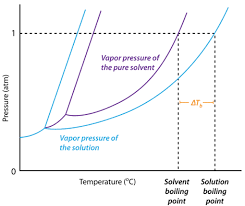## How to Calculate and Solve for Van’t Hoff Factor, Ebullioscopic Constant, Molality and Boiling Point Elevation | The Calculator EncyclopediaThe image above represents boiling point elevation.

To compute for the boiling point elevation, three parameters are needed and these parameters are Van’t Hoff’s Factor (i), ebullioscopic constant (Kb) and Molality.

The formula for calculating boiling point elevation:

δTb = iKb x Molality

Where;
δTb = boiling point elevation
i = Van’t Hoff’s Factor
Kb = ebullioscopic constant
Molality

Let’s solve an example;
Find the boiling point elevation when the Van’t Hoff’s Factor is 42, ebullioscopic constant is 60 and molality of 180.

This implies that;
i = Van’t Hoff’s Factor = 42
Kb = ebullioscopic constant = 60
Molality = 180

δTb = iKb x Molality
δTb = (42)(60) x 180
δTb = (2520) x 180
δTb = 453600

Therefore, the boiling point elevation is 453600 °C m-1.

Calculating the Molality using the Boiling Point Elevation, Van’t Hoff’s Factor and Ebullioscopic Constant.

Molality = δTb / iKb

Where;
Molality
δTb = boiling point elevation
i = Van’t Hoff’s Factor
Kb = ebullioscopic constant

Let’s solve an example;
Find the molality with a boiling point elevation of 120 and a van’t hoff’s factor of 32 with a ebullioscopic constant of 12.

This implies that;
δTb = boiling point elevation = 120
i = Van’t Hoff’s Factor = 32
Kb = ebullioscopic constant = 12

Molality = δTb / iKb
Molality = 120 / 384
Molality = 0.3125

Therefore, the molality is 0.3125.